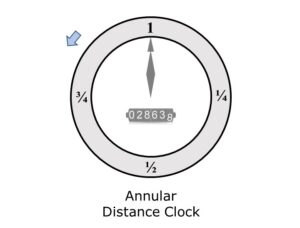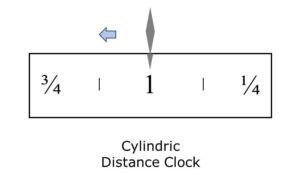iSoul In the beginning is reality.

# Distance clock

A time clock is a device that measures a constant rate of internal motion. Time clocks are synchronized to a common event and rate of internal motion. A time clock is used by correlating its internal measure with other motions and events. There is a constant relationship between a time clock and a distance clock, so in a sense they are interchangeable.

A distance (or length) clock, also called an odologe, is a device that measures a constant rate of external motion. Distance clocks are synstancialized to a common event and rate of external motion. A distance clock is used by correlating its external measure with other motions and events. A consistent distance clock requires a uniform motion at a fixed rate.Above is a distance clock illustrated: note that the pointer (hand) does not move, and the ring (face) rotates counter-clockwise so that the distances ¼, ½, ¾, and 1 unit of distance appear in order at the top. The odometer keeps a running total of the distance. The actual circumference need not match the unit distance; only the rate of motion as symbolized must match the unit rate. This means that smaller clocks would turn slower, and larger clocks would turn faster.In practice a distance in time is often perceived by the distance covered or distance remaining on a trip, with the rate of travel being a typical rate. One can measure the progress on a trip by either the time or distance.

Revised 2020-11-07